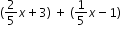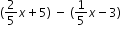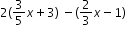# MAFS.7.EE.1.1Archived StandardExport Print
Apply properties of operations as strategies to add, subtract, factor, and expand linear expressions with rational coefficients.
General Information
Subject Area: Mathematics
Domain-Subdomain: Expressions & Equations
Cluster: Level 1: Recall
Cluster: Use properties of operations to generate equivalent expressions. (Major Cluster) -

Clusters should not be sorted from Major to Supporting and then taught in that order. To do so would strip the coherence of the mathematical ideas and miss the opportunity to enhance the major work of the grade with the supporting clusters.

Date of Last Rating: 02/14
Status: State Board Approved - Archived
Assessed: Yes
Test Item Specifications

• Assessment Limits :
Expressions must be linear and contain a variable.
• Calculator :

Neutral

• Context :

Allowable

Sample Test Items (3)
• Test Item #: Sample Item 1
• Question:

What is the sum of the two expressions?• Difficulty: N/A
• Type: EE: Equation Editor

• Test Item #: Sample Item 2
• Question:

Find the difference of the two expressions.• Difficulty: N/A
• Type: EE: Equation Editor

• Test Item #: Sample Item 3
• Question:

An expression is shown.Create an equivalent expression without parentheses.

• Difficulty: N/A
• Type: EE: Equation Editor

## Related Courses

This benchmark is part of these courses.
1205020: M/J Accelerated Mathematics Grade 6 (Specifically in versions: 2014 - 2015, 2015 - 2020, 2020 - 2022, 2022 and beyond (current))
1205040: M/J Grade 7 Mathematics (Specifically in versions: 2014 - 2015, 2015 - 2022, 2022 and beyond (current))
1204000: M/J Foundational Skills in Mathematics 6-8 (Specifically in versions: 2014 - 2015, 2015 - 2022, 2022 and beyond (current))
7812020: Access M/J Grade 7 Mathematics (Specifically in versions: 2014 - 2015, 2015 - 2018, 2018 - 2019, 2019 - 2022, 2022 and beyond (current))
7912115: Fundamental Explorations in Mathematics 2 (Specifically in versions: 2013 - 2015, 2015 - 2017 (course terminated))

## Related Access Points

Alternate version of this benchmark for students with significant cognitive disabilities.

## Related Resources

Vetted resources educators can use to teach the concepts and skills in this benchmark.

## Formative Assessments

Equivalent Perimeters:

Students are asked to solve a geometric problem by simplifying an algebraic expression.

Type: Formative Assessment

Equivalent Rational Expressions:

Students are given a polynomial with rational coefficients and asked to identify equivalent expressions from a given list.

Type: Formative Assessment

Factored Forms:

Students are given two expressions and asked to rewrite each in factored form using the fewest number of terms.

Type: Formative Assessment

Identify Equivalent Multistep Expressions:

Students are given an expression and are asked to identify expressions equivalent to it.

Type: Formative Assessment

## Lesson Plans

Gather Data For Distribution by Programming an App:

This lesson allow students to gather, calculate, and plot data using both computer code and mathematical equations. In this lesson students will create a pedometer app to demonstrate the understanding of algorithms, components (such as buttons, textboxes, sensors, etc.), and If/Then statements. This lesson uses algebraic equations and random data to access the needed components to store data in a spreadsheet.

Type: Lesson Plan

Data Sets Represented in Computers:

This lesson shows how data can be represented by computers, in relation to everyday activities we may not be aware that we use computer. It gives an overview of graphing data by creating a histogram based on population data. Using the data collected, students will get a chance to hand write code to show what structure is needed for computers to collect, analyze and distribute such data. This lesson is lesson 1 of the Data Set and Deviation Statistics Unit and bridges statistical concepts of data collection, graphing and analysis with programming a computer using coding language while reinforcing foundational algebraic skills.

Type: Lesson Plan

Using Positive and Negative Numbers in Context:

This lesson unit is intended to help you assess how well students are able to understand and use directed numbers in context. It is intended to help identify and aid students who have difficulties in ordering, comparing, adding, and subtracting positive and negative integers. Particular attention is paid to the use of negative numbers on number lines to explore the following structures:

• starting temperature + change in temperature = final temperature
• final temperature – change in temperature = starting temperature
• final temperature – starting temperature = change in temperature

Type: Lesson Plan

Steps to Solving Equations:

This lesson unit is intended to help you assess how well students are able to form and solve linear equations involving factorizing and using the distributive law. In particular, this unit aims to help you identify and assist students who have difficulties in using variables to represent quantities in a real-world or mathematical problem and solving word problems leading to equations of the form px + q = r and p(x + q) = r.

Type: Lesson Plan

Total Recall:

Using the notion of a broken robot, this lesson provides opportunities for students to apply different strategies and properties to expand, add, subtract, or multiply to determine equivalent expressions. The students will analyze their procedures and answers.

Type: Lesson Plan

Equivalent Expressions?:

Students are asked to determine if two expressions are equivalent and explain their reasoning.

Miles to Kilometers:

In this task students are asked to write two expressions from verbal descriptions and determine if they are equivalent. The expressions involve both percent and fractions. This task is most appropriate for a classroom discussion since the statement of the problem has some ambiguity.

## Tutorials

Factor a Linear Expression by Taking a Common Factor:

This video demonstrates how to factor a linear expression by taking a common factor.

Type: Tutorial

Combining Like Terms Introduction:

This introductory video teaches about combining like terms in linear equations.

Type: Tutorial

Solving Equations With the Variable on Both Sides.:

This video models solving equations in one variable with variables on both sides of the equal sign.

Type: Tutorial

## Unit/Lesson Sequence

Variables and Patterns of Change: Translating Words Into Symbols; Linear Equations:

Lesson Plan 1: Miles of Tiles - The Pool Border Problem, students will recognize patterns and represent situations using algebraic notation and variables. Lesson Plan 2: Cups and Chips - Solving Linear Equations Using Manipulatives, students use manipulatives to represent visually the steps they take to obtain a solution to an algebraic equation. They develop an understanding of the connections between the solution involving manipulatives and the symbolic solution. Students work in teams of four. Site includes a Topic Overview, Lesson Plans, Student Work, Teaching Strategies, Resources, and a video of Workshop 1; Part 1.

Type: Unit/Lesson Sequence

## MFAS Formative Assessments

Equivalent Perimeters:

Students are asked to solve a geometric problem by simplifying an algebraic expression.

Equivalent Rational Expressions:

Students are given a polynomial with rational coefficients and asked to identify equivalent expressions from a given list.

Factored Forms:

Students are given two expressions and asked to rewrite each in factored form using the fewest number of terms.

Identify Equivalent Multistep Expressions:

Students are given an expression and are asked to identify expressions equivalent to it.

## Student Resources

Vetted resources students can use to learn the concepts and skills in this benchmark.

Equivalent Expressions?:

Students are asked to determine if two expressions are equivalent and explain their reasoning.

Miles to Kilometers:

In this task students are asked to write two expressions from verbal descriptions and determine if they are equivalent. The expressions involve both percent and fractions. This task is most appropriate for a classroom discussion since the statement of the problem has some ambiguity.

## Tutorials

Factor a Linear Expression by Taking a Common Factor:

This video demonstrates how to factor a linear expression by taking a common factor.

Type: Tutorial

Combining Like Terms Introduction:

This introductory video teaches about combining like terms in linear equations.

Type: Tutorial

Solving Equations With the Variable on Both Sides.:

This video models solving equations in one variable with variables on both sides of the equal sign.

Type: Tutorial

## Parent Resources

Vetted resources caregivers can use to help students learn the concepts and skills in this benchmark.

Equivalent Expressions?:

Students are asked to determine if two expressions are equivalent and explain their reasoning.

Miles to Kilometers:

In this task students are asked to write two expressions from verbal descriptions and determine if they are equivalent. The expressions involve both percent and fractions. This task is most appropriate for a classroom discussion since the statement of the problem has some ambiguity.